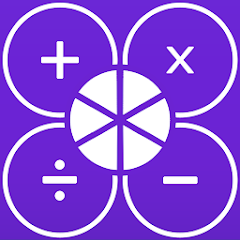# Fraction calculator XL

100+Everyone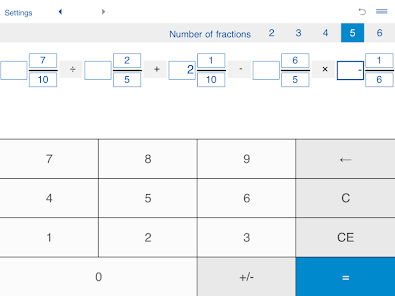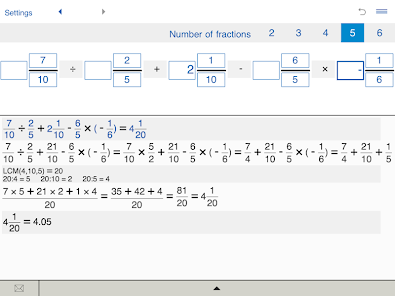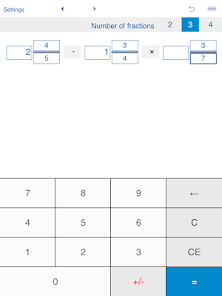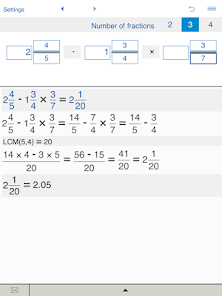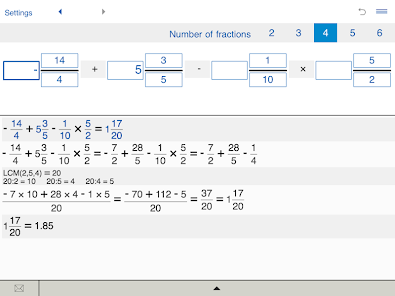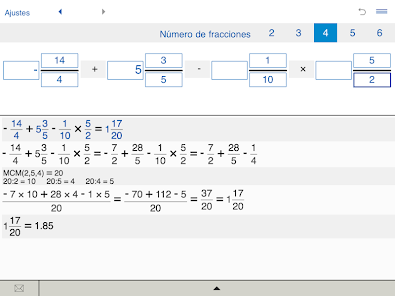The best app for understanding, learning, teaching and calculating fractions!

Fraction calculator XL is an easy-to use fraction calculator with steps that enables you to quickly solve fraction problems and makes learning fractions much easier.

Fraction calculator XL will help you quickly solve your homework problems or check your results.

Fraction calculator with steps to add, subtract, multiply, and divide fractions, whole and mixed numbers.

Features:

Fraction calculator for arithmetic operations with two, three, four, five, and six fractions: adding fractions, subtracting fractions, multiplying fractions, dividing fractions.

Fractions calculator helps you solve problems with fractions (proper and improper fractions), mixed numbers (mixed fractions), and whole numbers.

Addition and subtraction of fractions and mixed fractions with like and unlike denominators.

Basic calculations: addition, subtraction, multiplication and division.

Multiplication and division of fractions and mixed numbers.

Finds the least common denominator.

Supports negative values.

Fraction solver shows the details of calculation.

History tape to view your recent calculations.

Back and forward buttons to check or recall recent calculations.

Sends results and history via email.

'Undo' for the Clear command.
Updated on
Jul 30, 2020

## Data safety

Developers can show information here about how their app collects and uses your data. Learn more about data safetyNo information available